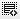Module 33: NPV0
In the Quiz question:
If you invested Rs. 10 lakhs (1 million) in a project and expect to get a steady cash flow of Rs. 1 lakhs (100,000) every year over the next 5 years. What is the NPV of this project assuming prevailing interest rates of 15%?

As per my calculations the
PV = 372,454.36 and
NPV = (627,545.64)

Whereas the Option for the right answer is (664,784)

Could you tell me as to where I am going wrong please.

Thanks.0

Hi supriya,

As per the formula for PV for the first year it should be 1lakh/(1+.15) = 86.95K
So going on like this

1year = 86.95K
2year = 75.60K
3Year = 65.74K
4Year = 57K
5Year = 50K
Adding these all will give you present value = 3.36Lakh
Net Present Value = 10Lakh - 3.36Lakh = 6.64Lakh which is there in the option.

Hope you got it!

Click on thisicon to add code snippet.